## Rotational Motion Exam2 and Problem Solutions

Rotational Motion Exam2 and  Problem Solutions

1. An object in horizontal rotates on a circular road with 10m/s velocity. It does 120 revolutions in one minute.

a) Find frequency and period of the object.

b) Find the change in velocity vector when it rotates 600, 900 and 1800.

a) 60s.f=120 revolution

f=2 revolution/second

T=1/f=1/2s

b)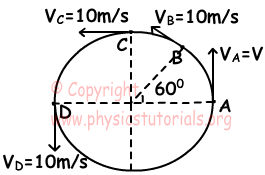If object starts its motion from point A and rotates 600 and comes point B;

ΔV=VB-VA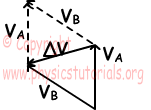ΔV=2V.cos1200/2=2.10.cos600=20/2=10m/s

If object rotates 900;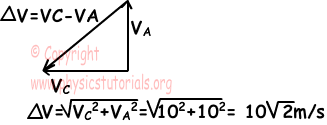If object rotates 1800;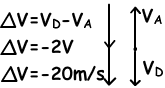2. A device does 1800 revolution in 6 minutes. Find angular velocity and period of this device. (π=3)

1800.T=6.60s

T=360/1800=1/5s

3. Friction constant of a circular road, having radius 50m, is 0,2. Find the initial velocity of the car having 1500 kg to turn junction safely.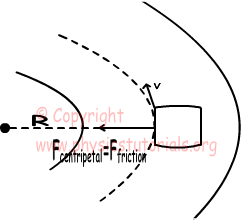Ffriction≥Fcentripetal (for safe turn)

k.m.g≥m.V2/r

k.g.r≥V2

100≥V2

10m/s≥V or

36km/h≥V

4. A marble is thrown with velocity V from point A. If no force is exerted on surface by marble at point B, find the force exerted on point A by marble. (Assume that velocity of marble is constant.)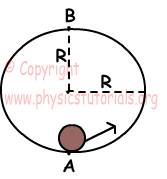Since no force is exerted on point B by marble;

mg=mV2/R

V2=g.R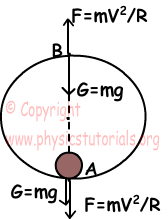Force exerted by marble on point A;

FA=G+F

FA=mg+mV2/R (we put V2=gR into the equation)

FA=mg+mgR/R

FA=2mg

5. Two objects rotate with same frequency around point O. One of the objects has mass m and other one has mass 9m. If centripetal forces exerted on objects having mass m is F1, and object having mass 9m is F2, find ratio of F1/F2.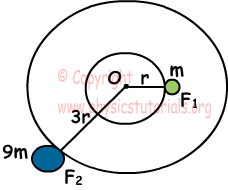V1=2.π.r.f

V2=2.π.3r.f

V2=3V1

F1/F2=mV12/r/9mV22/3r=V12/r.3r/9V22

F1/F2=1/27

Author: